# SAT Math Multiple Choice Question 817: Answer and Explanation

### Test Information

Question: 817

7.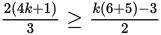Which of the following correctly describes the possible values of k in the inequality above?

• A.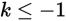• B.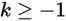• C.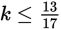• D.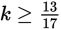Explanation:

C

Difficulty: Medium

Category: Heart of Algebra / Inequalities

Strategic Advice: Although cross-multiplication is an appropriate strategy when you have a fractional expression on each side of the equal sign, simplifying the numerators first will save a few steps and avoid some potential errors.

Getting to the Answer: Start by distributing the 2 on the left side and by adding 6 + 5 on the right side. Then cross-multiply to eliminate the denominators. Finally, solve for k using inverse operations. Don't forget-if you multiply or divide by a negative number at any point during the process, you must reverse the inequality symbol: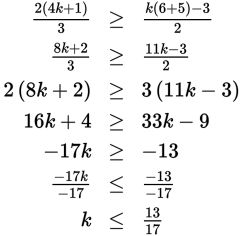(C) is correct.